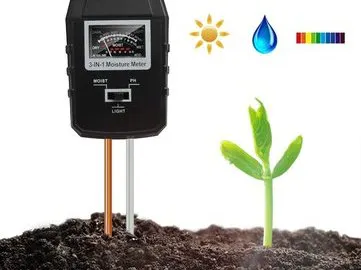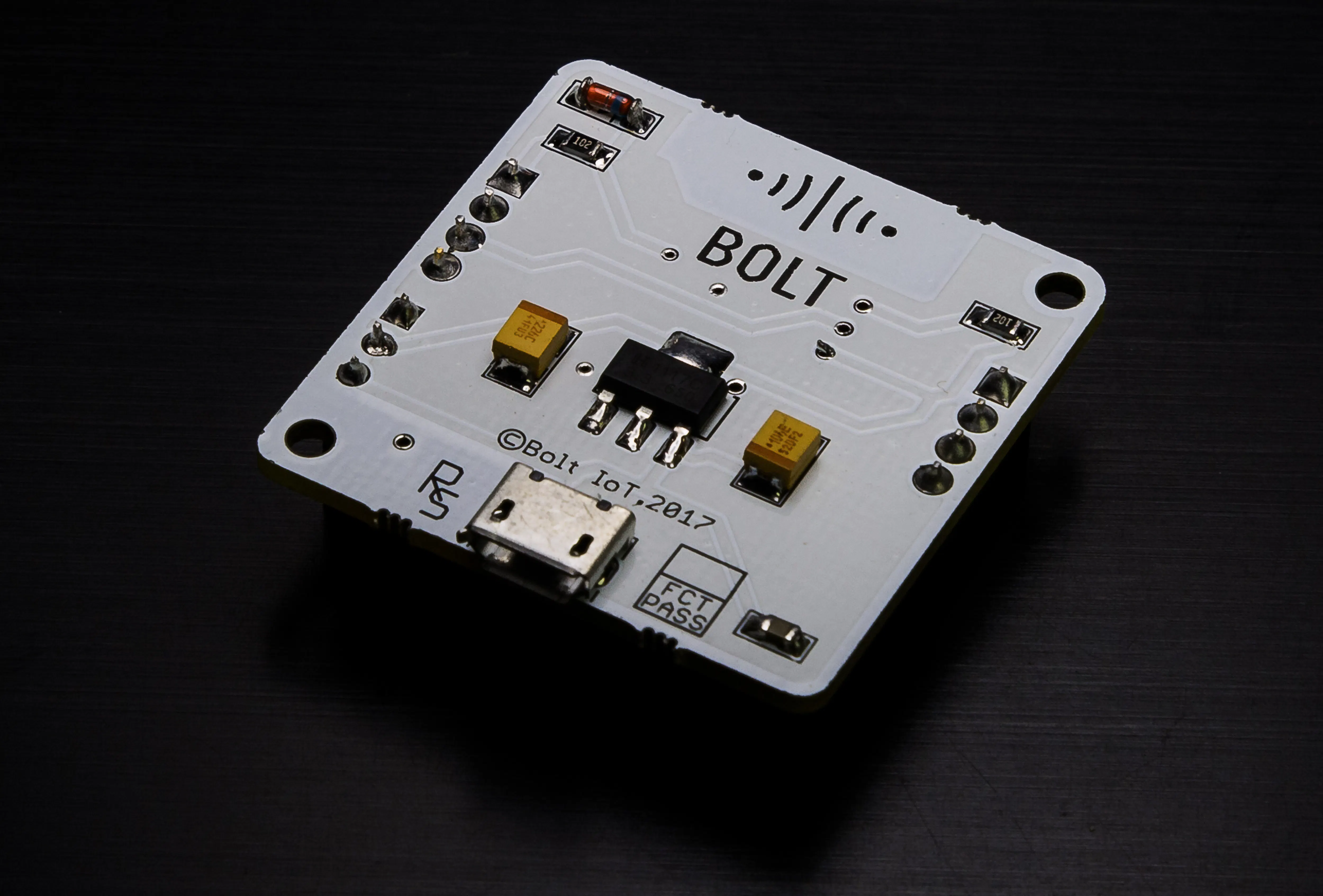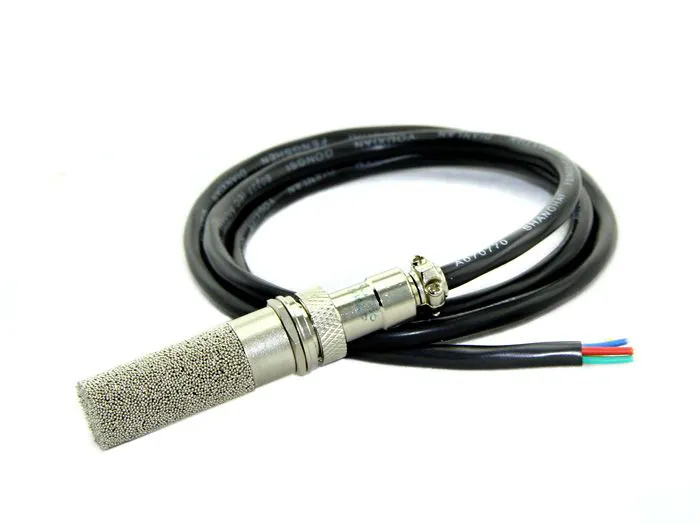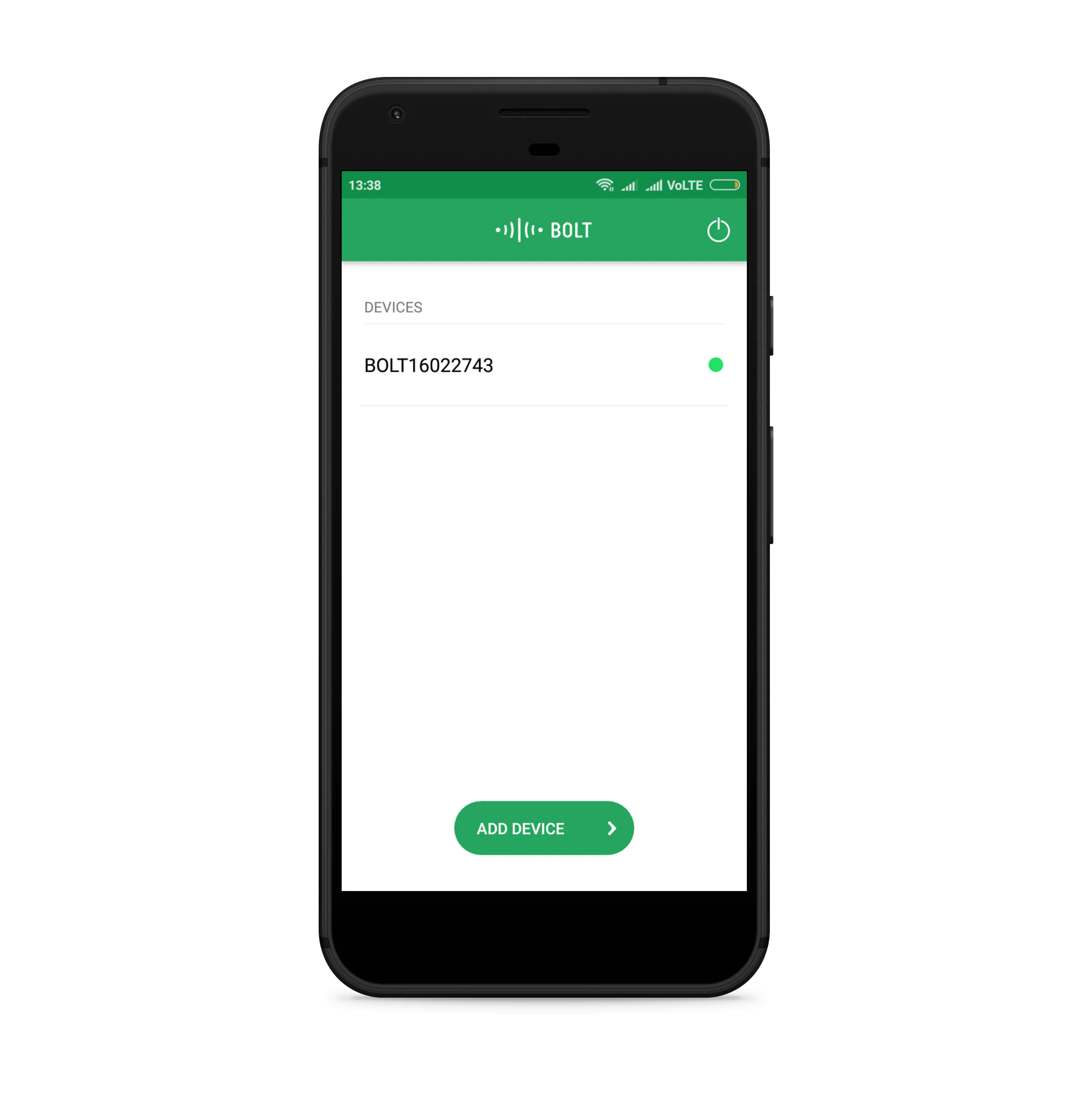# Soil Moisture Monitoring with Bolt IoT

Using YL-69/HL-69 sensor with the Bolt Wi-Fi Module to read the moisture of soil and send an SMS alert crossing threshold value.

BeginnerFull instructions provided4 hours92## Things used in this project

### Hardware componentsBolt IoT Bolt WiFi Module
×1Seeed Soil Moisture & Temperature Sensor
×1Jumper wires (generic)
×1USB-A to Mini-USB Cable
×1

### Software apps and online servicesBolt IoT Bolt CloudBolt IoT Android App

## Code

### soil moisture monitering code

Python
```import config    #this file contains the SSID API Key and Bolt device ID
import json,time,math,statistics    #used to call methods to fetch data...calculate Z cross....and give delays
from boltiot import Sms,Bolt     #used to call methods to sen SMS and use Bolt WiFi Module

def compute_bounds(history_data,frame_size,factor):       #This function was created to calculate the Mean,Variance and Z crosss
if len(history_data)<frame_size:
return None

if len(history_data)>frame_size:
del history_data[0:len(history_data)-frame_size]

Mn=statistics.mean(history_data)
Variance=0

for data in history_data :
Variance += math.pow((data-Mn),2)

Zn = factor * math.sqrt(Variance / frame_size)
High_bound = history_data[frame_size-1]+Zn
Low_bound = history_data[frame_size-1]-Zn

return [High_bound,Low_bound]        #This function returns a Upper Bound Threshold and Lower Bound Threshold

mybolt = Bolt(config.API_KEY, config.DEVICE_ID)        #To configure the Bolt WiFi Module
sms = Sms(config.SSID, config.AUTH_TOKEN, config.TO_NUMBER, config.FROM_NUMBER)       #To configure the Twilio SMS Service
history_data=[]           # This is a list to store the values recieved from the Sensor

while True:
data = json.loads(response)     #store the response given recieved in JSON format
if data['success'] != 1:            # To detect if the value recieved contains keyword success and corresponding value should be 1 denoting STATUS OK
print("There was an error while retriving the data.")
print("This is the error:"+data['value'])
time.sleep(10)
continue

print ("This is the value "+data['value'])  #To print the Analog Value received form the Bolt WiFi Module

try:
soil=(int(data['value'])/1024)*100   #To convert the vales in Percentage
soil = 100-soil	     #To find the moisture content left in soil out of total 100%
print ("The Moisture content is ",soil," % mg/L")
except e:
print("There was an error while parsing the response: ",e)
continue

bound = compute_bounds(history_data,config.FRAME_SIZE,config.MUL_FACTOR)

if not bound:
required_data_count=config.FRAME_SIZE-len(history_data)
print("Not enough data to compute Z-score.Need",required_data_count,"more data points")
history_data.append(soil)
time.sleep(10)
continue
try:
if soil < 30:
print ("The Moisture level has decreased. Sending an SMS.")
response = sms.send_sms("Please water the plants")
print("This is the response ",response)

except e:
print("There was an error while parsing the response: ",e)
continue

try:
if soil > bound :
print ("The Moisture level increased suddenly. Sending an SMS.")
response = sms.send_sms("Someone is damaging the plants")
print("This is the response ",response)
elif soil < bound:
print ("The Moisture level decreased suddenly. Sending an SMS.")
response = sms.send_sms("Someone is damaging the plants")
print("This is the response ",response)

print("HIGH BOUND = ",bound)
print("LOW BOUND = ",bound)
history_data.append(soil)

except Exception as e:
print ("Error",e)
time.sleep(10)
```

### config.py

Python
```SSID = 'xxxxxx'
AUTH_TOKEN = 'xxxxxx'
FROM_NUMBER = '+xxxxx'
TO_NUMBER = '+91xxxx'
API_KEY = 'xxxxxxx'
DEVICE_ID = 'xxxxxx'
FRAME_SIZE = 10
MUL_FACTOR = 3
```

## Credits

### Vallabh deshpande

1 project • 1 follower